# Volume of Rectangular Prisms Lesson Plan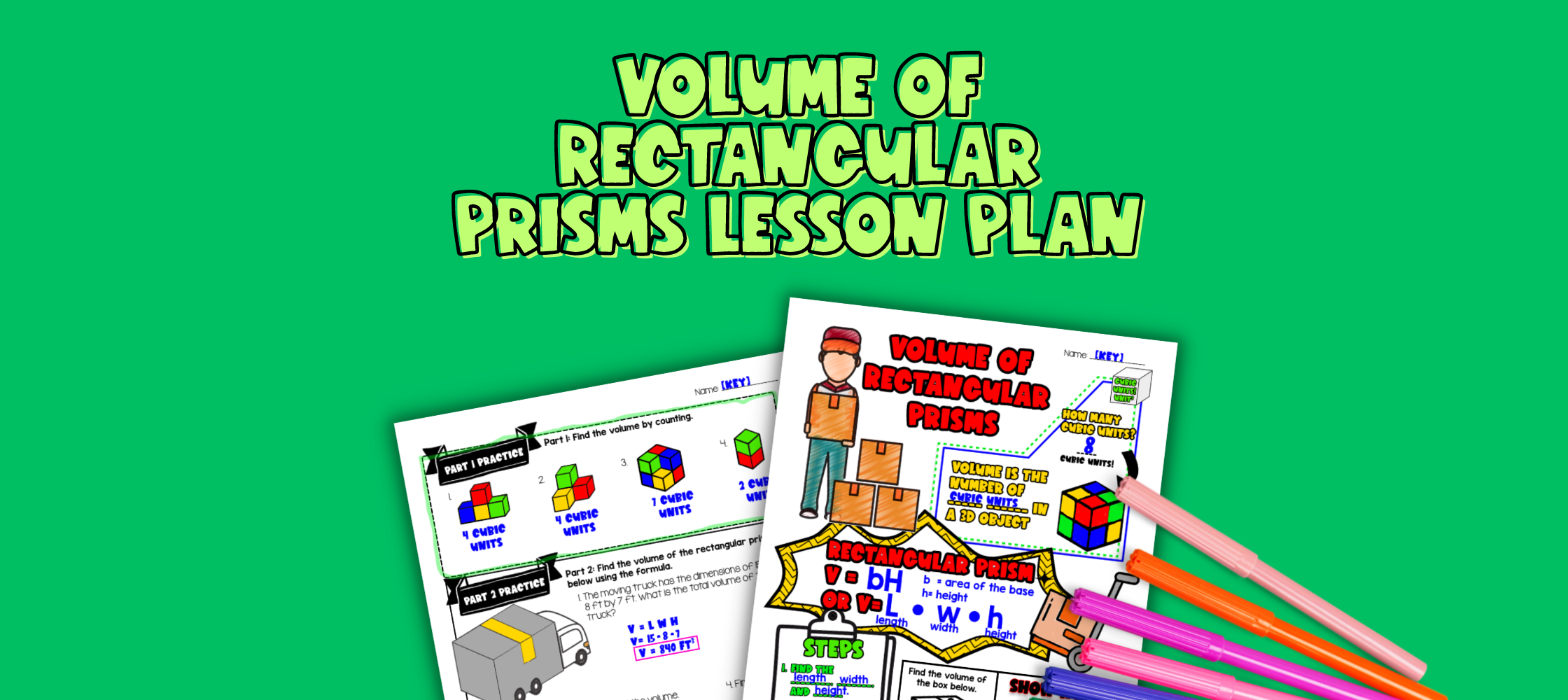Pin This

## Overview

Have you ever wondered how to teach volume of rectangular prism to your 6th graders? Or struggled with explaining which two formulas can you use to find volume of rectangular prisms and why are these two formulas equivalent?

Use this artistic, real-life lesson plan to teach your students about volume of rectangular prisms. Students will learn material with guided notes (like sketch notes) and practice with a doodle and color by number activity and a maze activity to cover the concept.

Then, it concludes with a real life application of volume for moving to a new city. Students learn about the importance of calculating the volume of rectangular prisms to determine what size moving truck you need for all your boxes.

## Get the Lesson Materials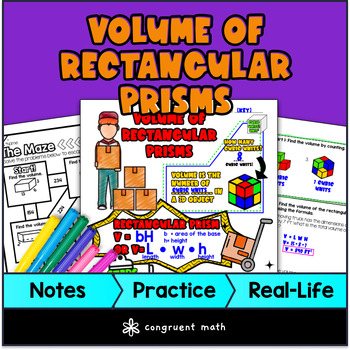\$4.25

## Learning Objectives

After this lesson, students will be able to:

• Find the volume of rectangular prisms by counting

• Use the formula to find the volume of rectangular prisms

• Apply the concept of volume of rectangular prisms in real-life situations, such as calculating the necessary truck size for a move

Note: This just covers volume of rectangular prisms. For surface area, see my Surface Area of Rectangular Prisms lesson plan. For other shapes, see my Volume of Cylinders, Cones, Spheres lesson plan.

## Prerequisites

Before this lesson, students should be familiar with:

• Basic multiplication and division skills of whole numbers and decimals

• Understanding of the concept of area

• Familiarity with basic geometry terms, such as width, height, and length

## Key Vocabulary

• Rectangular prism

• Volume

• Base

• Length, Width, Height

• Cubic units

## Procedure

### Introduction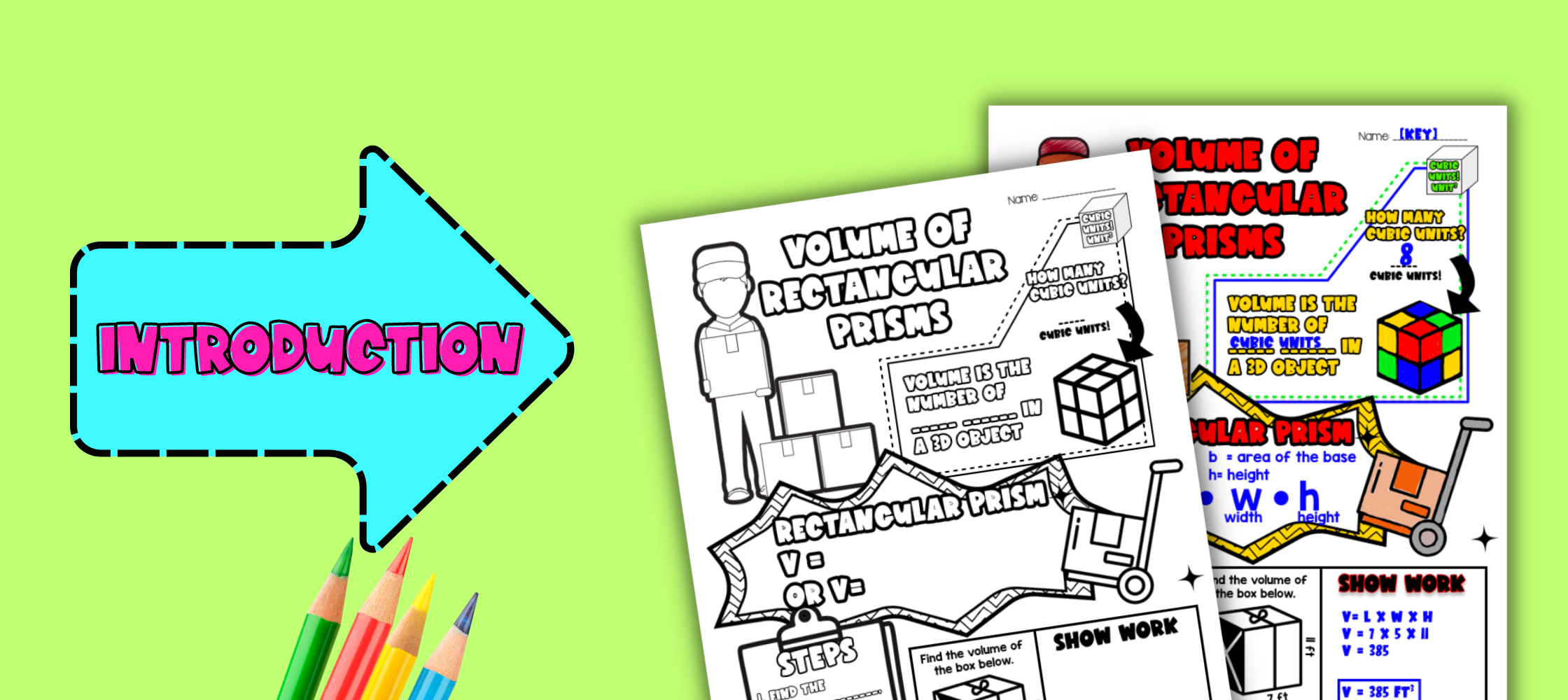Pin This
• As a hook, ask students whether they’ve ever planned a move before, and how they decided what size truck they needed. Pivot into a discussion of rectangular prisms. Refer to the real-life application section of the lesson plan for ideas.

• Use the guided notes to introduce the concept of volume of rectangular prisms. Walk through the key points of the topic of the guided notes to teach. Refer to the FAQs below for a walk through on this, as well as ideas on how to respond to common student questions.

### Practice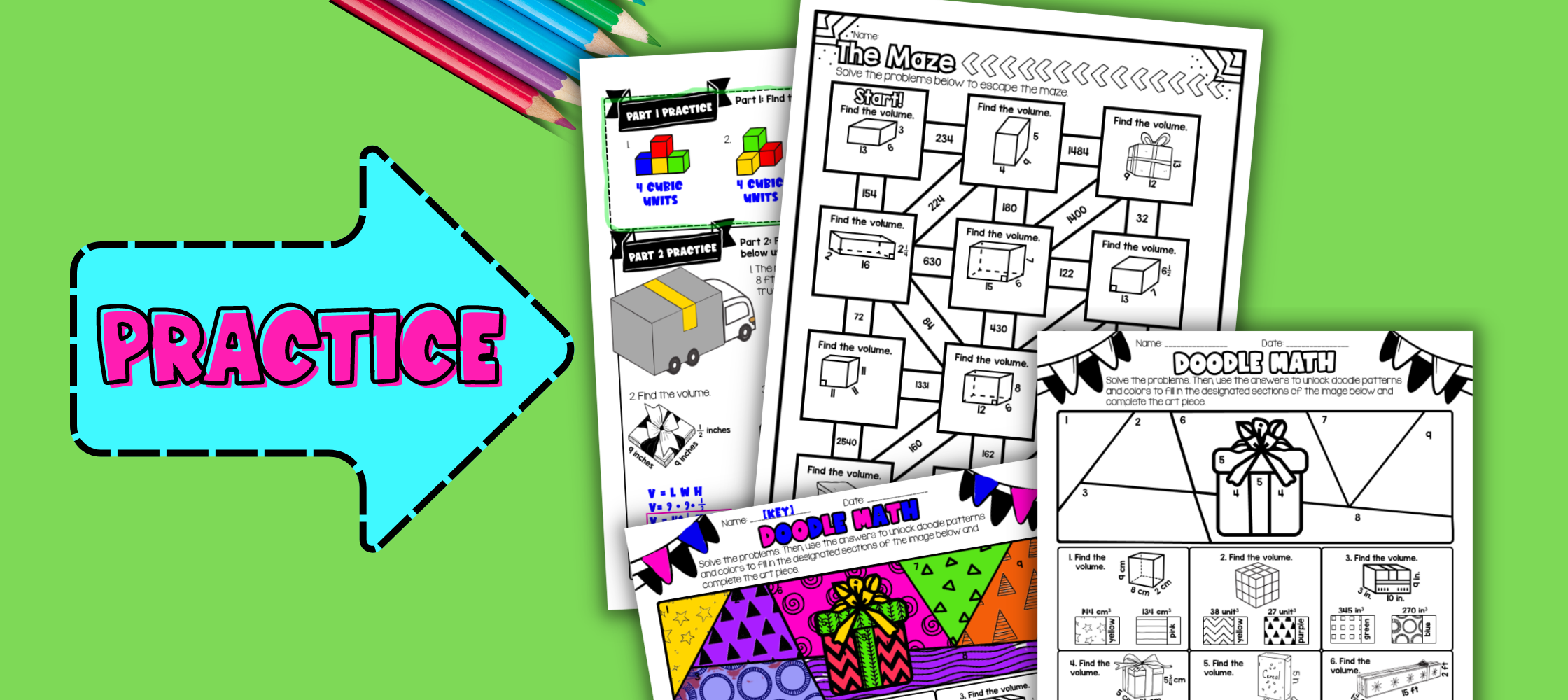Pin This
• Have students work through the problems on practice page, either collaboratively or independently. Walk around and spot-check student answers on the check for understanding activity, or call on students around answers they’ve picked and have students come up to the whiteboard and demonstrate.

• The practice page is divided into two sections, volume by counting, and volume by formula. This split gives a moment to break to discuss the answers of one problems before moving on.

• Based on student responses, reteach concepts that students need extra help with. If your class has a wide range of proficiency levels, you can pull out students for reteaching, and have more advanced students begin work on the practice exercises.

• Have students practice finding the volume of rectangular prisms using the Doodle Math activity. Walk around to answer student questions.

• Fast finishers can dive into the maze activity for extra practice. You can assign it as homework for the remainder of the class.

### Real-Life Application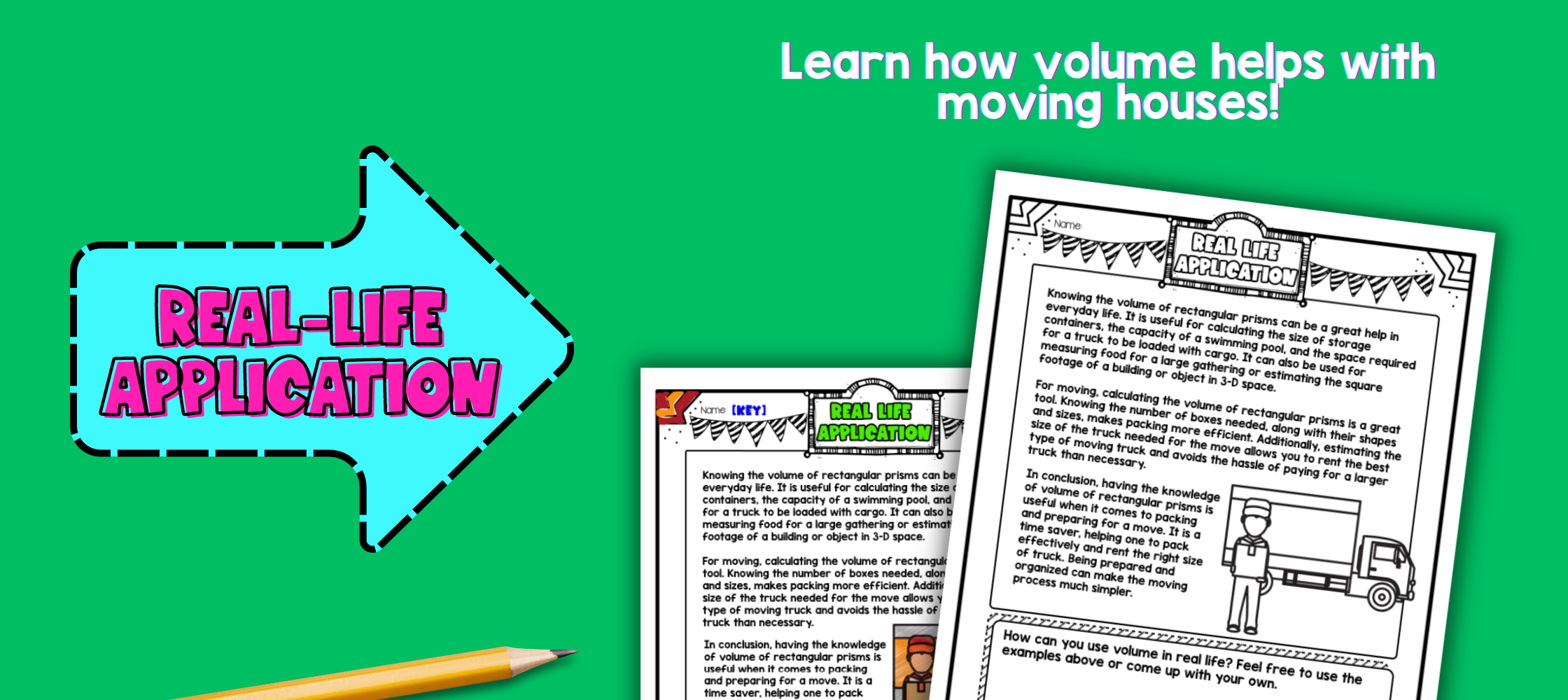Pin This
• Bring the class back together, and introduce the concept of calculating the volume of rectangular prisms when packing boxes for a move. Discuss how it's important to choose the right size truck, so you don't end up with too little space or waste money on a truck that's too big. Have students brainstorm other real-life situations where it might be important to calculate the volume of a rectangular prism, such as building a bookshelf or filling a fish tank. Refer to the FAQs for more ideas on how to teach it.

## Extensions

### Hands-on project

If you’re looking for a way for your kinesthetic learners to engage with 3D shape nets, why not have them build emoji and Minecraft characters from 3D shape nets?

There’s different versions depending on your students’ needs:

• Whole numbers. Practice finding surface area and volume of 3D shape nets with whole numbers, and end the activity with a version of Steve!

• Fractions. Practice finding surface area and volume of 3D shape nets with whole numbers, and end the activity with a version of the Creeper.

You can also buy both as a bundle, and let students choose which to build.

## Want more ideas and freebies?

Get my free resource library with digital & print activities—plus tips over email.